# Reflection and Refraction

This routine computes losses due to optical effects at the module level, namely reflection and refraction losses on the sunny-side glass superstrate. This is known as the incidence angle modifier (IAM). Three models are supported: Sandia, ASHRAE, and Tabular.

# Sandia IAM

This model computes the incidence angle modifier coefficients based on the Sandia method.

## Outputs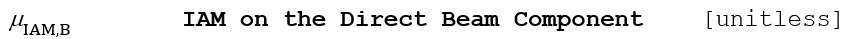## Algorithm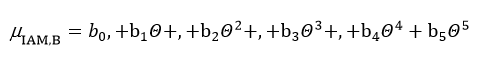Only the beam component ΘEff,D is treated here. Furthermore, it was observed that the best fit of the IAM coefficients for both FSLR anti-reflective coating (ARC) and regular non-ARC modules shows an oscillation below incidence angles of 34°, as shown in Figure 32.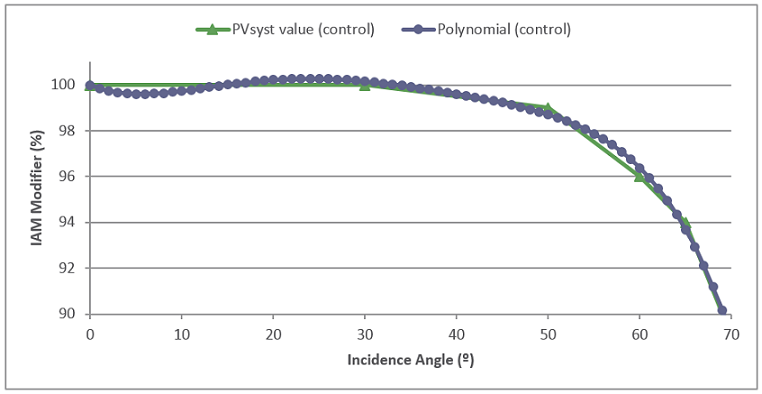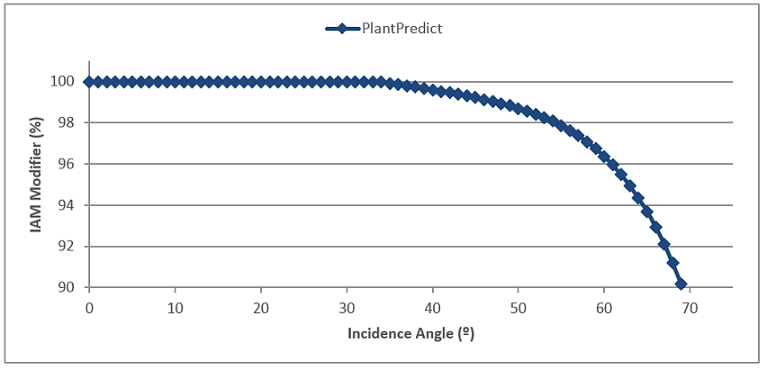### Figure 32. Comparison of Custom IAM Profile with Sandia Polynomial, Showing Oscillation for Incidence Angles Lower than 34°

To avoid this artifact, the polynomial is clipped to force the IAM to unity, as follows: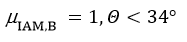## Reference

King, D. L., Kratochvil, J.A., Boyson, W.L., Measuring Solar Spectral and Angle-of-Incidence Effects on Photovoltaic Modules and Solar Irradiance Sensors. Sandia National Laboratories, Albuquerque, NM, September 1997.

# ASHRAE Direct Beam IAM

This model uses the incidence angle modifier coefficients based on the ASHRAE parameterization.

## Inputs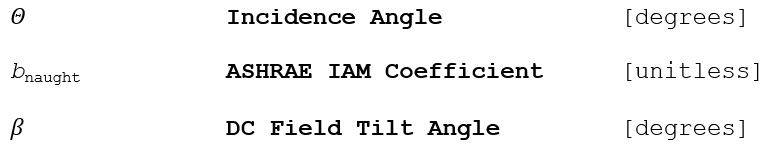## Outputs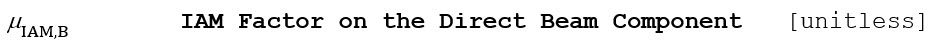## Algorithm (Direct Beam Component)

1.) Compute the direct beam incidence angle attenuation coefficient as a function of the incidence angle.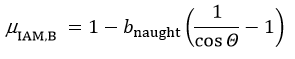2.) The incidence angle function approaches a singularity and is only valid at incidence angles less than 87°.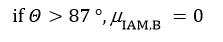3.) Reject all shading factors where the sun is found to be behind the plane of the array, i.e. the back of the module may be lit if the solar incidence angle is less than zero: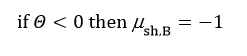The shading factor here is assigned to a value of -1 (in order to distinguish it from 0 (fully shaded) and 1 (unshaded). A negative shading factor does not have any effect on the irradiance and will not contribute to the “shading loss” calculation.

# Tabular IAM

This model uses incidence angle modifier coefficients defined in a table in the Module file.

## Inputs## Outputs## Algorithm (Direct Beam Component)

1.) Calculate the direct beam incidence angle modifier by doing a cubic spline interpolation on IAM tabular values.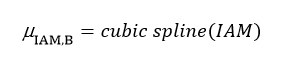2.) If μIAM,B is greater than 1, set it to 1.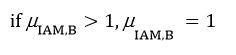# Diffuse IAM losses

Based on the work of Brandemuehl and Beckman (1980), the following equation integrates the effect of the Angle of Incidence (AOI) for all angles within the field of view to provide an overall AOI correction factor for the diffuse radiation, Fd: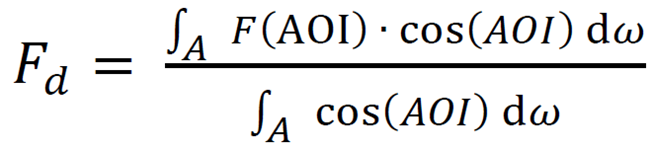where ω is the solid angle of the incident diffuse irradiance and A is the range of ω. The radiation’s F(AOI) is weighted by its contribution to the in-plane irradiance.

F(AOI) is in this case the tabular IAM values that are part of the module file. The equivalent numerical equation that is being used becomes: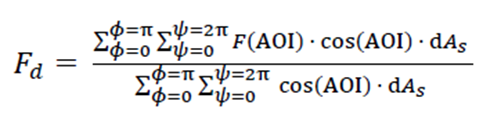Please see the below drawing for a more detailed explanation: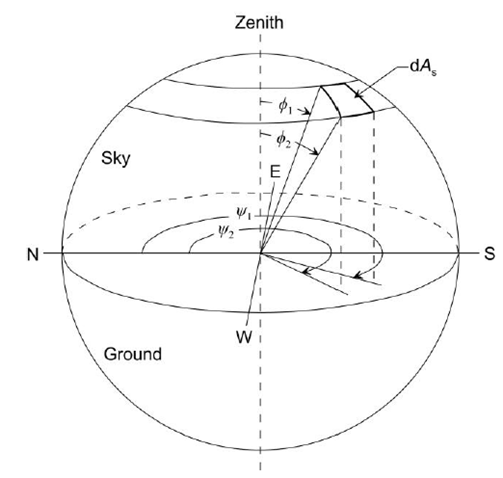Reference:

B. Marion “Numerical method for angle-of-incidence correction factors for diffuse radiation incident photovoltaic modules”, Solar Energy, Volume 147, Pages 344-348. 2017. DOI: 10.1016/j.solener.2017.03.027